Rounding (nearest 10 and 100) Math Video for Kids | 3rd, 4th, 5th Grade
1%
It was processed successfully!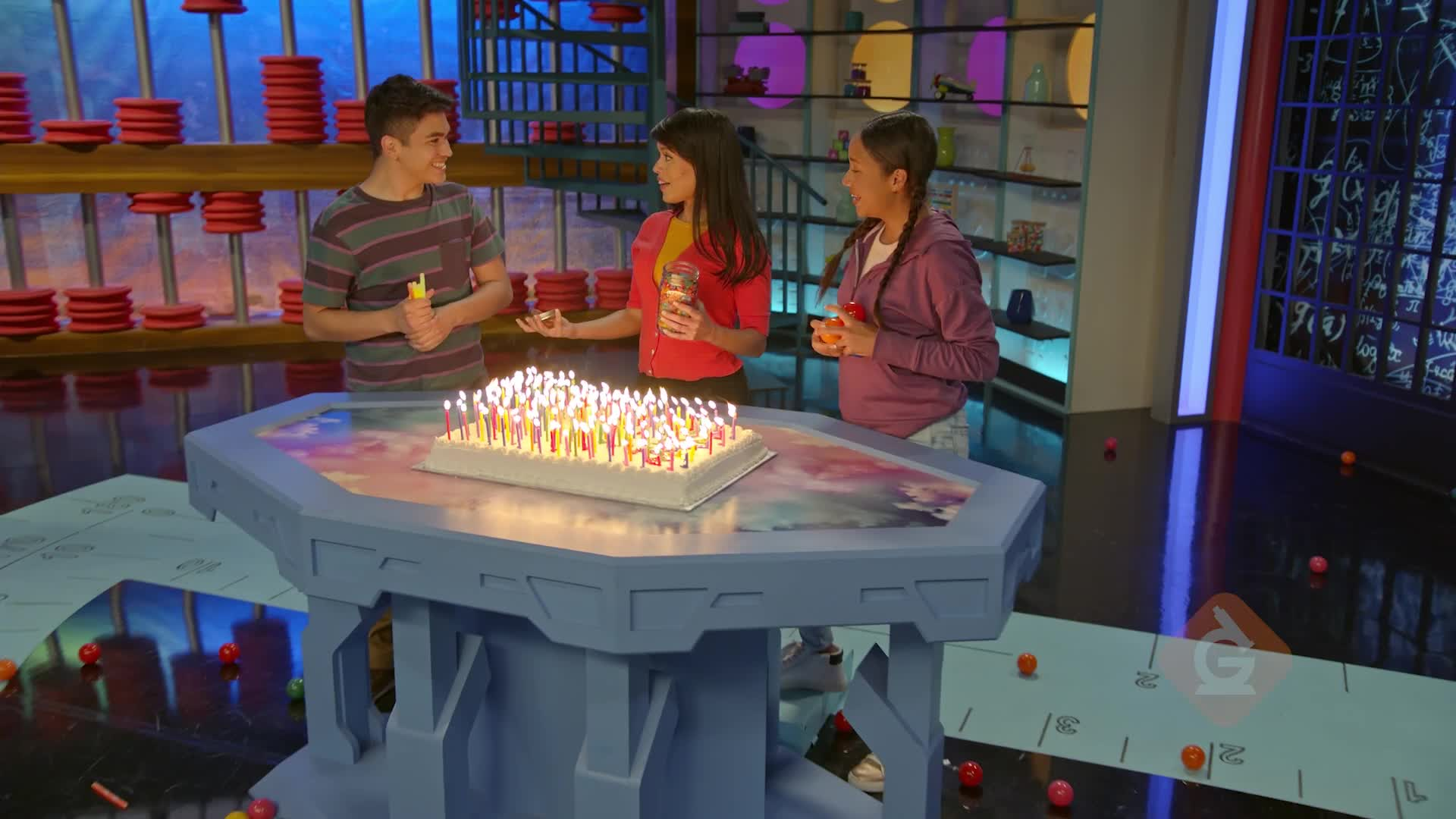WHAT IS ROUNDING TO THE NEAREST 10 AND 100?

Rounding means choosing a simpler number close to the original number. These simpler numbers may be easier to solve problems with.

To better understand Rounding (NEAREST 10 AND 100)…

WHAT IS ROUNDING TO THE NEAREST 10 AND 100?. Rounding means choosing a simpler number close to the original number. These simpler numbers may be easier to solve problems with. To better understand Rounding (NEAREST 10 AND 100)…

## LET’S BREAK IT DOWN!

### Toy Cars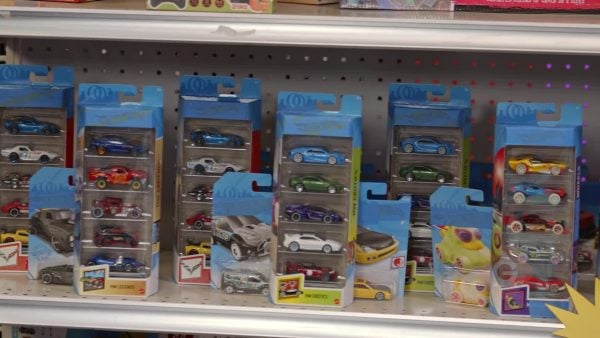Let’s say you have 57 toy cars and you want to round the number of cars you have to the nearest ten. You can draw a number line from 50 to 60 to help you round. After you draw the number line, mark the halfway point at 55. Then you can locate 57 on the number line. 57 is greater than 55 so you know that the point for 57 is to the right of 55. Now you can see that 57 is closer to 60 than to 50, so you round up to 60. You have about 60 toy cars. Try this one yourself. You bought 42 flowers for a party. Round the number of flowers you bought to the nearest ten.

Toy Cars Let’s say you have 57 toy cars and you want to round the number of cars you have to the nearest ten. You can draw a number line from 50 to 60 to help you round. After you draw the number line, mark the halfway point at 55. Then you can locate 57 on the number line. 57 is greater than 55 so you know that the point for 57 is to the right of 55. Now you can see that 57 is closer to 60 than to 50, so you round up to 60. You have about 60 toy cars. Try this one yourself. You bought 42 flowers for a party. Round the number of flowers you bought to the nearest ten.

### Bouncy Balls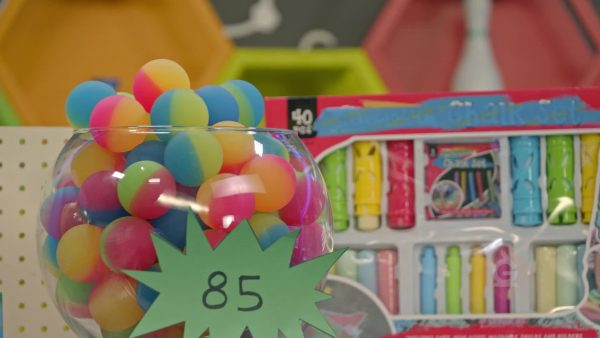Let’s say you have 85 bouncy balls and you want to round the number of bouncy balls you have to the nearest ten. 85 bouncy balls is between 80 and 90. You make a number line from 80 to 90 and mark the halfway point at 85. Since the number of bouncy balls you have is halfway between 80 and 90, you follow the rounding rule and round up to 90. You have about 90 bouncy balls. There is a pattern. You don’t have to always draw a number line. If you want to round to the nearest ten, you can look at the digit in the ones place. If it’s 4 or less, you round down. If it’s 5 or greater, you round up. Since there is a 5 in the ones place, you round up to 90 and get the same answer: about 90 bouncy balls. Try this one yourself. You have 64 bouncy balls. Round the number of bouncy balls you have to the nearest ten.

Bouncy Balls Let’s say you have 85 bouncy balls and you want to round the number of bouncy balls you have to the nearest ten. 85 bouncy balls is between 80 and 90. You make a number line from 80 to 90 and mark the halfway point at 85. Since the number of bouncy balls you have is halfway between 80 and 90, you follow the rounding rule and round up to 90. You have about 90 bouncy balls. There is a pattern. You don’t have to always draw a number line. If you want to round to the nearest ten, you can look at the digit in the ones place. If it’s 4 or less, you round down. If it’s 5 or greater, you round up. Since there is a 5 in the ones place, you round up to 90 and get the same answer: about 90 bouncy balls. Try this one yourself. You have 64 bouncy balls. Round the number of bouncy balls you have to the nearest ten.

### Cows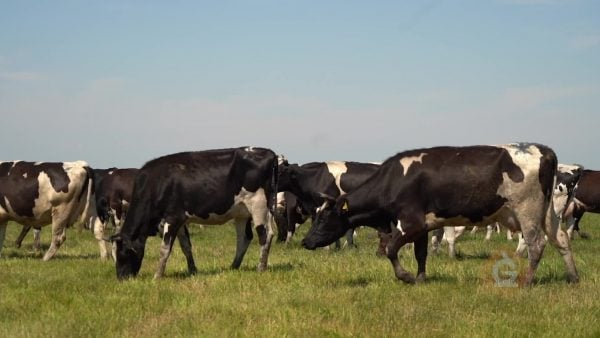Let’s say you’re a farmer and you have 142 cows. You want to round the number of cows you have to the nearest ten. To round to the nearest ten, look at the digit to the right of the tens place. If it’s 5 or more, you round up. If it’s 4 or less, you round down. 142 has a 2 in the ones place, so you round down to 140. You have about 140 cows. Try this one yourself. A farmer has 178 pigs. Round the number of pigs they have to the nearest ten.

Cows Let’s say you’re a farmer and you have 142 cows. You want to round the number of cows you have to the nearest ten. To round to the nearest ten, look at the digit to the right of the tens place. If it’s 5 or more, you round up. If it’s 4 or less, you round down. 142 has a 2 in the ones place, so you round down to 140. You have about 140 cows. Try this one yourself. A farmer has 178 pigs. Round the number of pigs they have to the nearest ten.

### Jelly Beans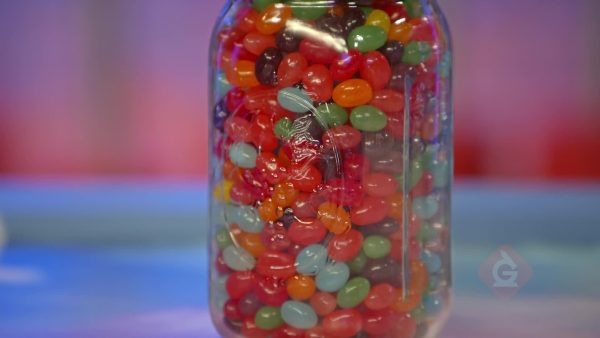Let’s say you have 329 jelly beans and you want to round the number of jelly beans you have to the nearest hundred. To round to the nearest hundred, look at the digit to the right of the hundreds place. If it’s 5 or more, you round up. If it’s 4 or less, you round down. 329 has a 2 in the tens place, so you round down to 300. You have about 300 jelly beans. Try this one yourself. You have 378 jelly beans. Round the number of jelly beans you have to the nearest hundred.

Jelly Beans Let’s say you have 329 jelly beans and you want to round the number of jelly beans you have to the nearest hundred. To round to the nearest hundred, look at the digit to the right of the hundreds place. If it’s 5 or more, you round up. If it’s 4 or less, you round down. 329 has a 2 in the tens place, so you round down to 300. You have about 300 jelly beans. Try this one yourself. You have 378 jelly beans. Round the number of jelly beans you have to the nearest hundred.

## ROUNDING (NEAREST 10 AND 100) VOCABULARY

Rounding
Makes numbers less exact but more convenient to work with.
Nearest ten
The multiple of ten that is closest to a given number.
Nearest hundred
The multiple of one hundred that is closest to a given number.
Number line
A straight line with numbers placed at equal intervals along its length.
Halfway point
A point that is halfway between two numbers on a number line.
Ones place
The place in a number that tells how many ones are in the number. In 247, there are 7 ones in the ones place.
The place in a number that tells how many tens are in the number. In 247, there are 4 tens in the tens place.
The place in a number that tells how many hundreds are in the number. In 247, there are 2 hundreds in the hundreds place.
Round to a number that is greater than the original number.
Round to a number that is less than the original number.

## ROUNDING (NEAREST 10 AND 100) DISCUSSION QUESTIONS

### How can you draw and label a number line to help you round 378 to the nearest ten?

Draw a number line from 370 to 380. Put a tick mark in the middle and label it 375. 378 is greater than 375, so plot the point for 378 between 375 and 380. 378 is closer to 380 than 370, so 378 rounds up to 380.

### What are the rules for rounding?

Look to the right of the place you want to round to. If the digit in that place is 5 or greater, round up. If the digit in that place is less than 5, round down.

### What steps would you go through to round 645 to the nearest ten using the rules?

First I look at the tens place, which has the digit 4. The place to the right of that place is the ones place, and it has a 5. When I have a 5 in the place to the right, I round up, so 645 rounded to the nearest ten is 650.

849.

257.
X

## Success

We’ve sent you an email with instructions how to reset your password.
Ok

## Choose Your Free Trial Period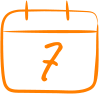### 7 DaysContinue to Lessons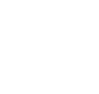### 90 DaysGet 90 days free by inviting other teachers to try it too.

Share with Teachers

## Get 90 Days Free

### By inviting 4 other teachers to try it too.

4 required

Skip, I will use a 7 days free trial

## Thank You!

Enjoy your free 90 days trial